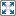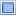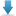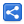# Java Ternary ?: operator - Numeric operands conversion rules

[Last Updated: Jun 3, 2017]

Java ternary expressions `(<boolean expression>? expr1: expr2)` are considered to be shorthand for if/else statement but they are not always equivalent and developers may have unexpected results in some cases. In this tutorial, we will go through numeric ternary expression type conversion rules (JLS 15.25.2) along with examples. We will also be quoting the statements from JLS 15.25.2.

## Rule 1: Same Type Rule

If the second and third operands have the same type, then that is the type of the conditional expression.

This is very straight forward rule:

` boolean b = true; int i = 5; int j = 7; int r = b ? j : i; System.out.println(r);`
``` 7
```
` boolean b2 = true; Double d1 = Double.valueOf(3); Double d2 = Double.valueOf(4); System.out.println(b2 ? d1 : d2);`
``` 3.0
```
` boolean b3 = true; Double d3 = null; Double d4 = Double.valueOf(4); System.out.println(b3 ? d3 : d4);`
``` null
```

## Rule 2: A primitive with it's boxed operand rule

If one of the second and third operands is of primitive type T, and the type of the other is the result of applying boxing conversion (§5.1.7) to T, then the type of the conditional expression is T.

That means, the ternary expressions evaluates to the primitive one, given that one operands is primitive and other one is it's boxed version. The unboxing of boxed type may be performed, depending whether the boolean part of ternary expression is true or false.

` boolean b = true; double op1 = 5d; Double op2 = 7d;//boxed double r = b ? op2 : op1; System.out.println(r);`
``` 7.0
```

But how do we know, in above example, that double r is the correct assigned type and we are not doing an unboxing on wrapper Double. Let's see another example to confirm that.

```public class Rule2Example {
public static void main(String[] args) {
boolean b = true;
double op1 = 5d;
Double op2 = 7d;//boxed
print(b ? op2 : op1);
}

private static void print(double d) {
System.out.println("primitive double");
System.out.println(d);
}

private static void print(Double d) {
System.out.println("boxed double");
System.out.println(d);
}
}```

#### Output

`primitive double7.0`

In above example the overloaded method with primitive double gets called (most specific method is selected), that confirms our ternary expression evaluates to primitive double and not to it's wrapper Double.

 Be careful of the NullPointerException:` boolean b = true; Integer i = null; int j = 5; System.out.println(b ? i : j);```` java.lang.NullPointerException at com.logicbig.example.Test.convert(Test.java:17) at com.logicbig.Common.feature.tasks.dynamic.CodeParser.lambda\$getHtmlOutput\$0(CodeParser.java:85) at com.logicbig.Common.code.CmdUtils.captureStdOutput(CmdUtils.java:19) ...... ```
 Above NPE happens because null value of Integer i, cannot be unboxed to primitive int. One way to fix above example is: ` boolean b = true; Integer i = null; int j = 5; System.out.println(b ? i : Integer.valueOf(j));```` null ```
 After above change, Rule 2 does not apply anymore, because both operands are of same type Integer now (rule 1 applies here).

## Rule 3: byte/Byte with short/Short rule

If one of the operands is of type byte or Byte and the other is of type short or Short, then the type of the conditional expression is short.

In this case result is always primitive short.

```public class Rule3Example {
public static void main(String[] args) {
boolean b = true;
short s = 2;
byte t = 3;
print(b ? t : s);

Short s2 = 4;
byte t2 = 5;
print(b ? t2 : s2);

short s3 = 6;
Byte t3 = 7;
print(b ? t3 : s3);

Short s4 = 8;
Byte t4 = 9;
print(b ? t4 : s4);
}

private static void print(byte b) {
System.out.println("primitive byte");
System.out.println(b);
}

private static void print(Byte b) {
System.out.println("Byte");
System.out.println(b);
}

private static void print(short s) {
System.out.println("primitive short");
System.out.println(s);
}

private static void print(Short s) {
System.out.println("Short");
System.out.println(s);
}
}```

#### Output

`primitive short3primitive short5primitive short7primitive short9`

## Rule 4: byte/short/char with constant int rule

If one of the operands is of type T where T is byte, short, or char, and the other operand is a constant expression (§15.28) of type int whose value is representable in type T, then the type of the conditional expression is T.

In this case result is same as of the primitive operand type (byte/short/char).

` boolean b = true; char c = 'a'; System.out.println(b ? 100 : c);`
``` d
```
In above example, the constant 100 is converted to char.

Other examples:

```public class Rule4Example {
public static void main(String[] args) {
boolean b = true;
byte t = 2;
short s = 3;
char c = 'z';
print(b ? 120 : t);
print(b ? 120 : s);
print(b ? 120 : c);
}

private static void print(byte b) {
System.out.print("byte: ");
System.out.println(b);
}

private static void print(short s) {
System.out.print("short: ");
System.out.println(s);
}

private static void print(char c) {
System.out.print("char: ");
System.out.println(c);
}

private static void print(int i) {
System.out.print("int: ");
System.out.println(i);
}
}```

#### Output

`byte: 120short: 120char: x`

We should also understand that the target type of an assignment can change the final type:

` boolean b = true; char c = 'a'; int i = b ? 100 : c; System.out.println(i);`
``` 100
```
 Generally, a final left side conversion of the assignment, may be performed during runtime. In above example, during compile time, the right side is decided to be converted to 'char' (according the rule we are discussing). During runtime, real conversion happens and the ternary expression returns a 'char' and then the char is converted (one more time) to left side int. Same rule is applied at other places like the method return type or the method argument. Let's see what happens, if we assign it to an Object. In that case right side, which evaluates to char, will be boxed to Character. ` boolean b = true; char c = 'a'; Object o = b ? 100 : c; System.out.println(o); System.out.println(o.getClass());```` d class java.lang.Character ```

## Rule 5: Byte/Short/Character with constant int rule

If one of the operands is of type T, where T is Byte, Short, or Character, and the other operand is a constant expression of type int whose value is representable in the type U which is the result of applying unboxing conversion to T, then the type of the conditional expression is U.

That simply means, the result will be of the primitive int type. The unboxing of the Byte/Short/Character operand to an int value may be performed, depending whether boolean part of the ternary expression is true or false.

```public class Rule5Example {
public static void main(String[] args) {
boolean b = false;
Byte t = Byte.valueOf((byte) 2);
Short s = Short.valueOf((short) 3);
Character c = Character.valueOf('z');
print(b ? 120 : t);
print(b ? 120 : s);
print(b ? 120 : c);
}

private static void print(Byte b) {
System.out.print("Byte: ");
System.out.println(b);
}

private static void print(Short s) {
System.out.print("Short: ");
System.out.println(s);
}

private static void print(Character c) {
System.out.print("Character: ");
System.out.println(c);
}

private static void print(int i) {
System.out.print("int: ");
System.out.println(i);
}
}```

#### Output

`int: 2int: 3int: 122`

## Rule 6: The binary promotion rule

Otherwise, binary numeric promotion (§5.6.2) is applied to the operand types, and the type of the conditional expression is the promoted type of the second and third operands.

That means, if none of the above Rule 1 to Rule 5 applies then ternary expression will be evaluated to a type which can be assignable to both numeric operands. A widening primitive conversion may also be applied.

` boolean b = true; int i = 4; double d = 5; System.out.println(b ? i : d);`
``` 4.0
```
 In above example the int value of 4 is promoted to double, that's because 'double' type is the one which is assignable to both 'int' and 'double'. More examples: ` boolean b = true; char c = 't'; double d = 5; System.out.println(b ? c : d);```` 116.0 ```
 An unboxing of a Number wrapper may be performed` boolean b = true; Short s = 2; float f = 5; System.out.println(b ? s : f);```` 2.0 ```

All primitives:

```public class Rule6Example {

public static void main(String[] args) {
boolean b = true;
byte t = 1;
Short s = 2;
int i = 3;
long l = 4;
float f = 6;
double d = 7;

print(b ? t : l);
print(b ? t : f);
print(b ? s : l);
print(b ? i : f);
print(b ? l : f);
print(b ? s : d);
print(b ? i : l);
}

private static void print(byte v) {
System.out.print("primitive byte, ");
System.out.println(v);
}

private static void print(short s) {
System.out.print("primitive short: ");
System.out.println(s);
}

private static void print(int v) {
System.out.print("primitive int: ");
System.out.println(v);
}

private static void print(long v) {
System.out.print("primitive long: ");
System.out.println(v);
}

private static void print(float v) {
System.out.print("primitive float: ");
System.out.println(v);
}

private static void print(double v) {
System.out.print("primitive double: ");
System.out.println(v);
}

private static void print(Object v) {
System.out.printf("wrapper : %s, ", v.getClass());
System.out.println(v);
}
}```

#### Output

`primitive long: 1primitive float: 1.0primitive long: 2primitive float: 3.0primitive float: 4.0primitive double: 2.0primitive long: 3`

Primitives and boxed type mixed (the result is always of primitive type):

```public class Rule6Example2 {

public static void main(String[] args) {
boolean b = true;
Byte t = 1;
Short s = 2;
int i = 3;
Integer i2 = 4;
Long l = 5L;
Float f = 6F;
Double d = 7d;
Double d2 = 8d;

print(b ? t : l);
print(b ? t : f);
print(b ? s : l);
print(b ? i : f);
print(b ? l : f);
print(b ? s : d);
print(b ? i : l);
print(b ? i2 : l);
print(b ? f : l);
print(b ? f : d);
print(b ? d2 : d);//it's rule 1
}
.............
}```

#### Output

`primitive long: 1primitive float: 1.0primitive long: 2primitive float: 3.0primitive float: 5.0primitive double: 2.0primitive long: 3primitive long: 4primitive float: 6.0primitive double: 6.0wrapper : class java.lang.Double, 8.0`

## Example Project

Dependencies and Technologies Used:

• JDK 1.8
• Maven 3.3.9

 Ternary Numeric Expression ExamplesSelect AllDownload• ternary-examples
• src
• main
• java
• com
• logicbig
• example
• Rule2Example.java
 Share